Скачать презентацию Objectives Objectives cont Post-2004 numbering

666c103fbc9ecda41d28b222684bc03f.ppt

• Количество слайдов: 51ObjectivesObjectives (cont. )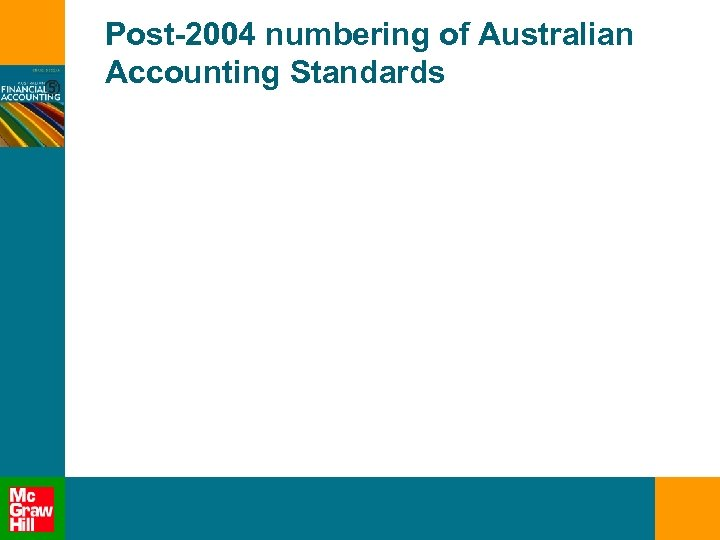Post-2004 numbering of Australian Accounting Standards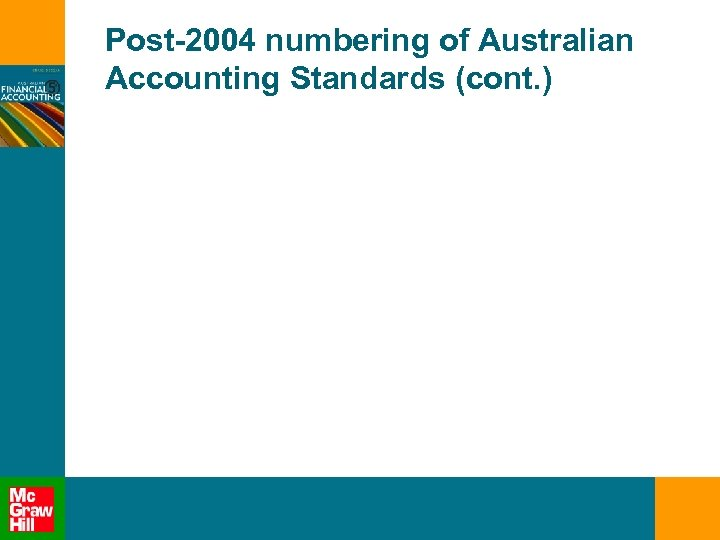Post-2004 numbering of Australian Accounting Standards (cont. )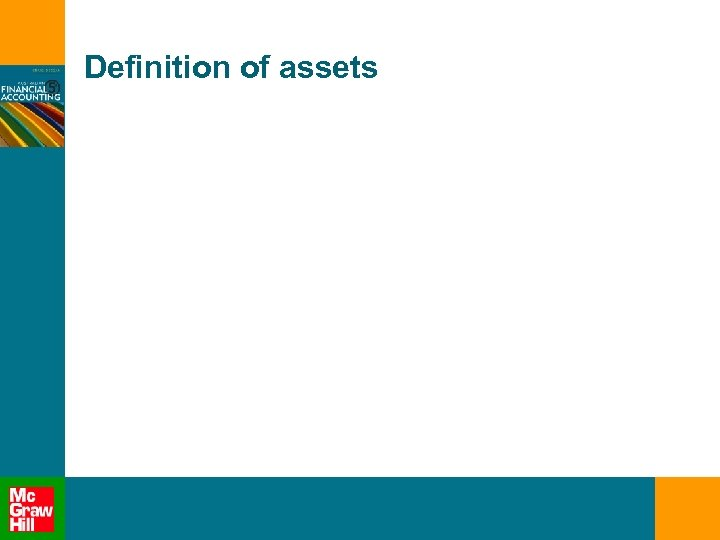Definition of assetsDefinition of assets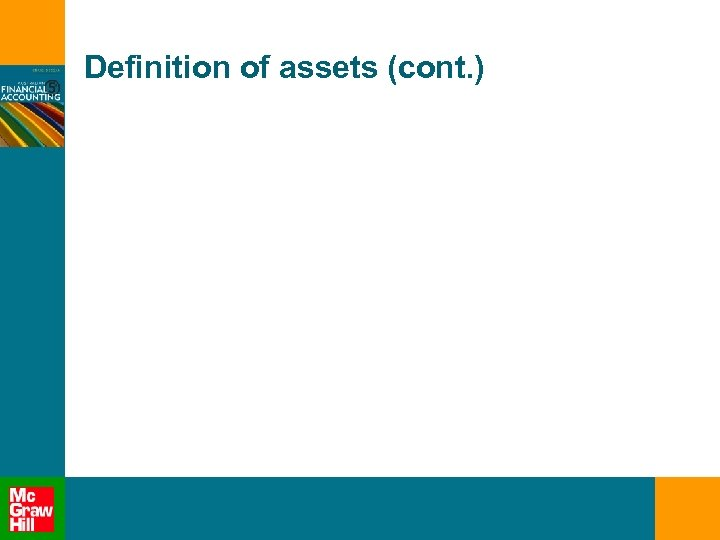Definition of assets (cont. )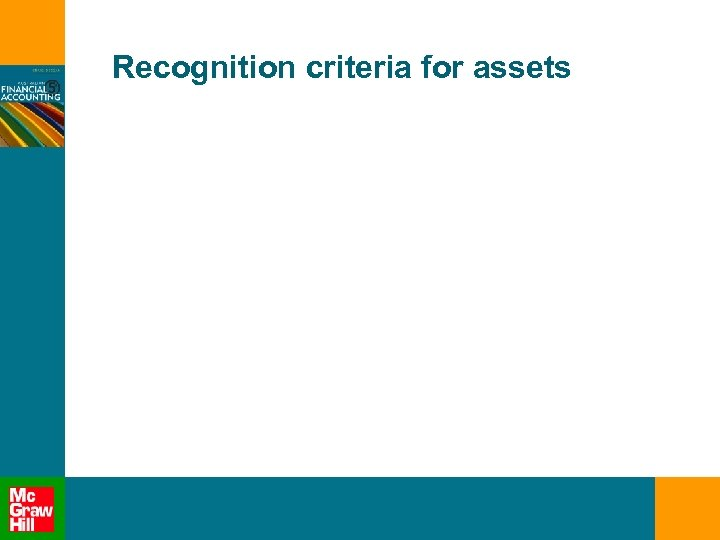Recognition criteria for assets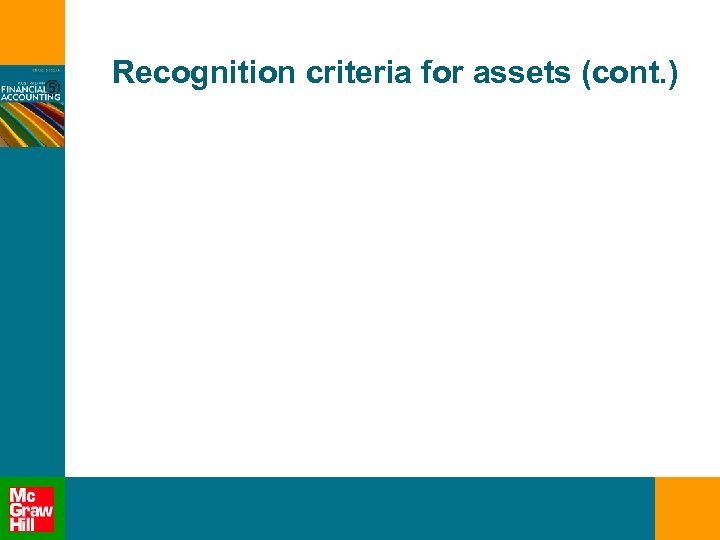Recognition criteria for assets (cont. )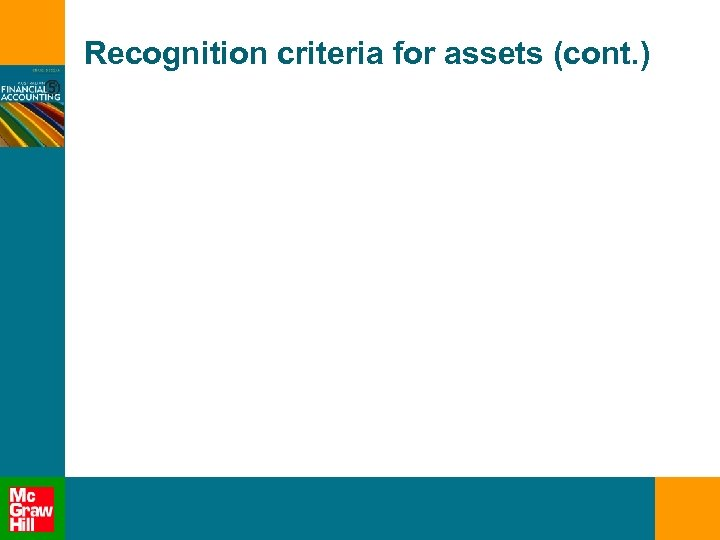Recognition criteria for assets (cont. )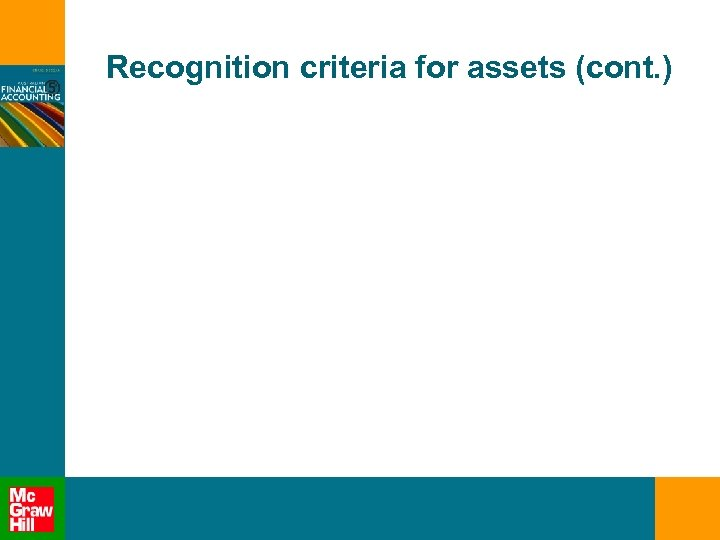Recognition criteria for assets (cont. )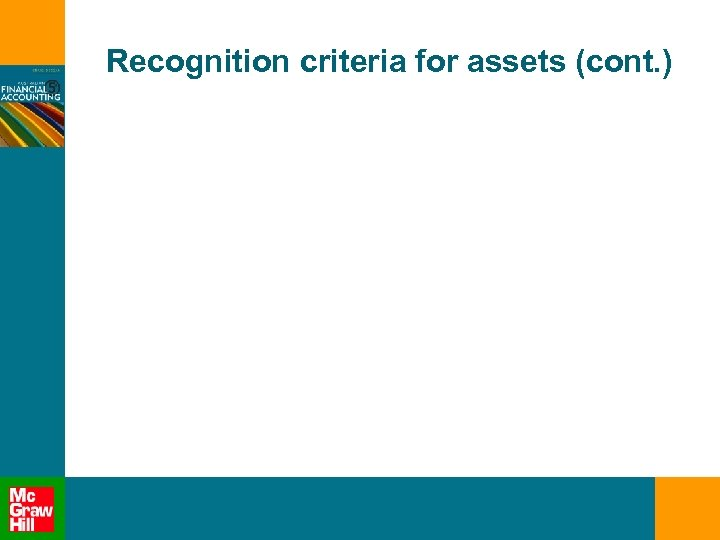Recognition criteria for assets (cont. )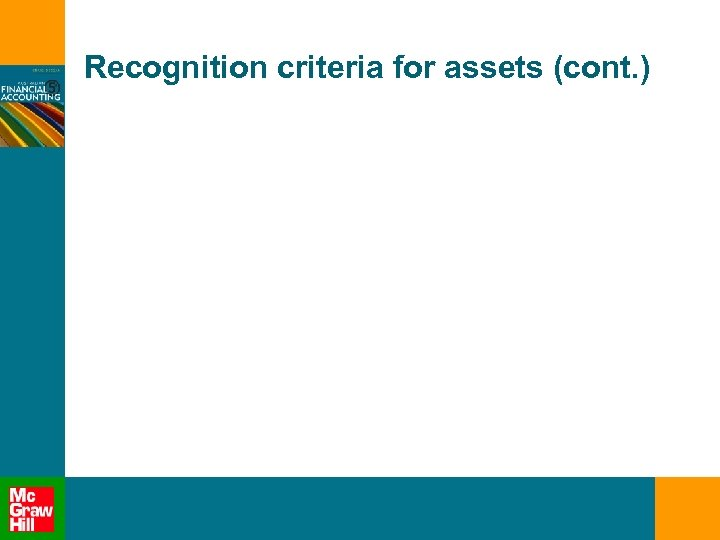Recognition criteria for assets (cont. )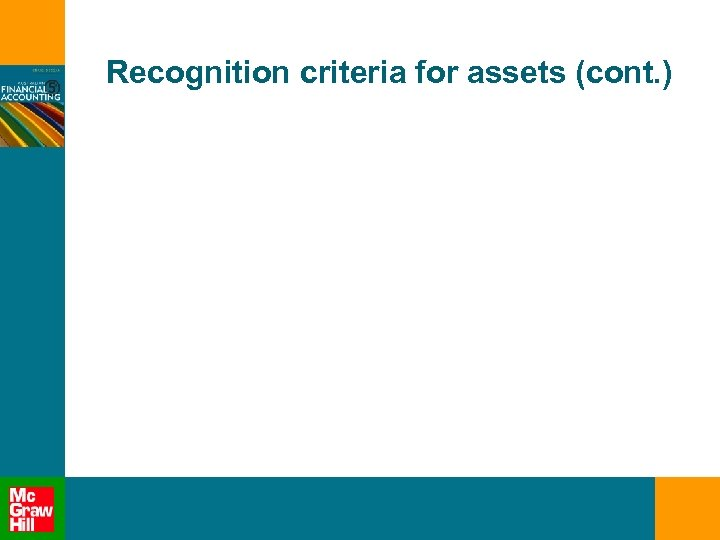Recognition criteria for assets (cont. )Recognition criteria for assets (cont. )Recognition criteria for assets (cont. )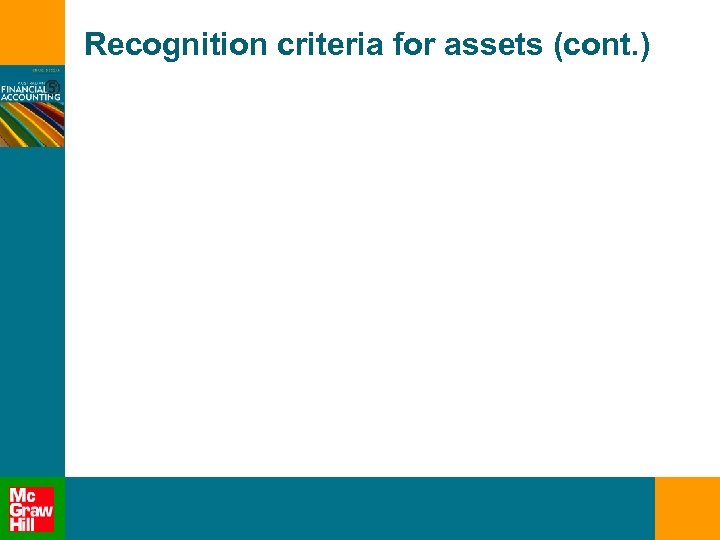Recognition criteria for assets (cont. )Recognition criteria for assets (cont. )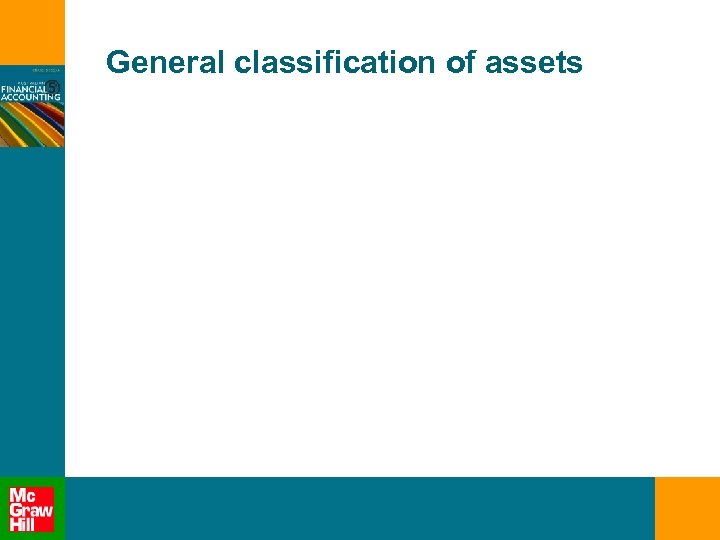General classification of assets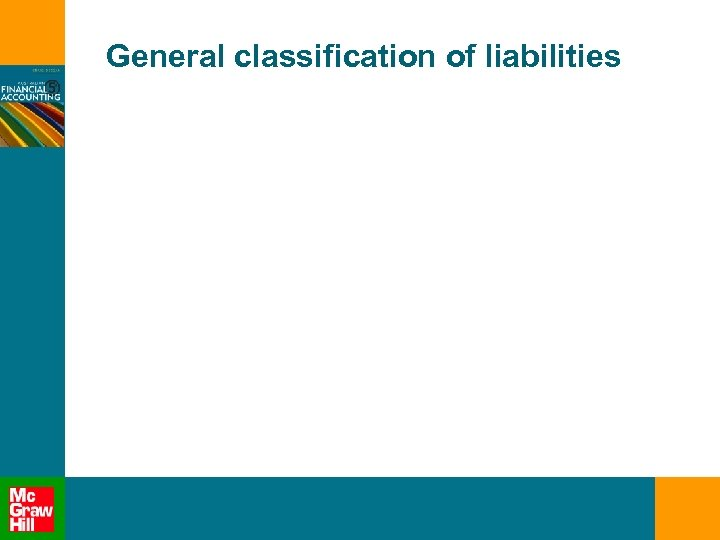General classification of liabilitiesHow to present a balance sheetHow to present a balance sheet (cont. )How to present a balance sheet (cont. )How to present a balance sheet (cont. )How to present a balance sheet (cont. )How to present a balance sheet (cont. )How to present a balance sheet (cont. )Determination of future economic benefitsDetermination of future economic benefits (cont. )Determination of future economic benefits (cont. )Determination of future economic benefits (cont. )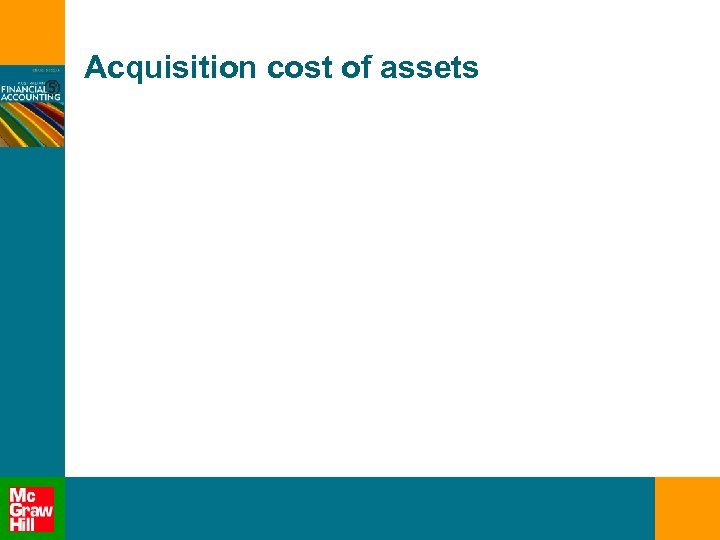Acquisition cost of assets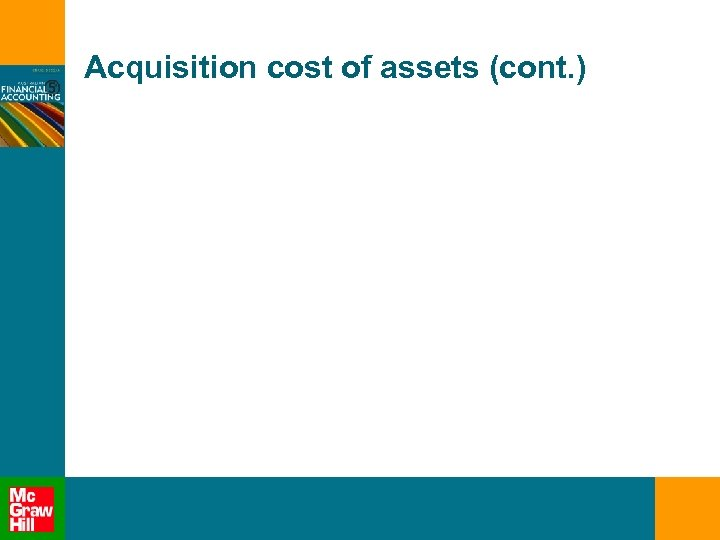Acquisition cost of assets (cont. )Acquisition cost of assets (cont. )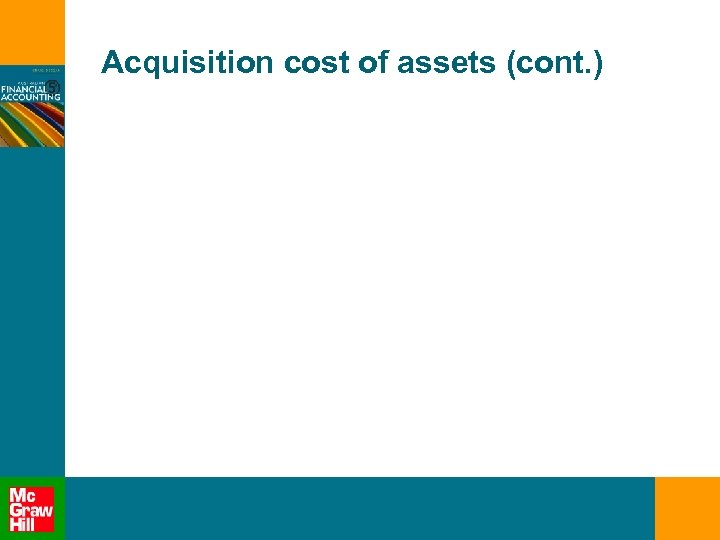Acquisition cost of assets (cont. )Acquisition cost of assets (cont. )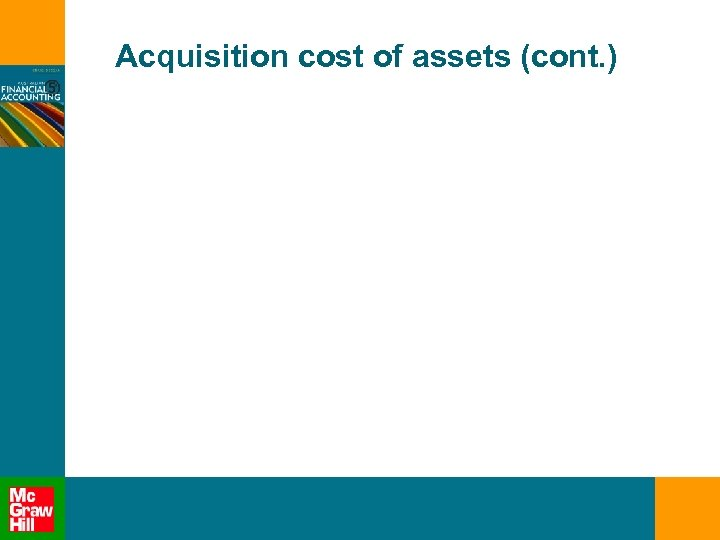Acquisition cost of assets (cont. )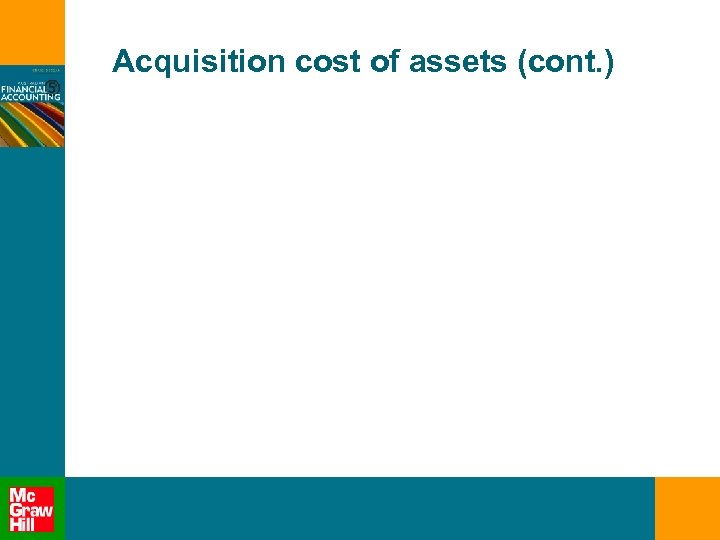Acquisition cost of assets (cont. )Acquisition cost of assets (cont. )Acquisition cost of assets (cont. )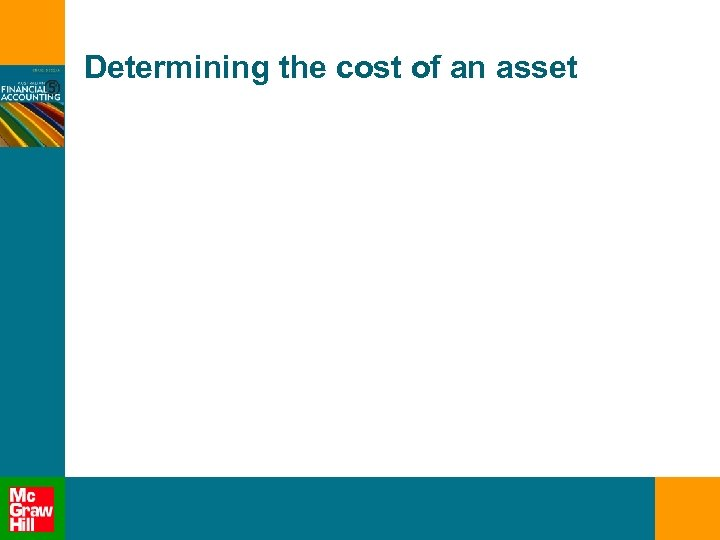Determining the cost of an asset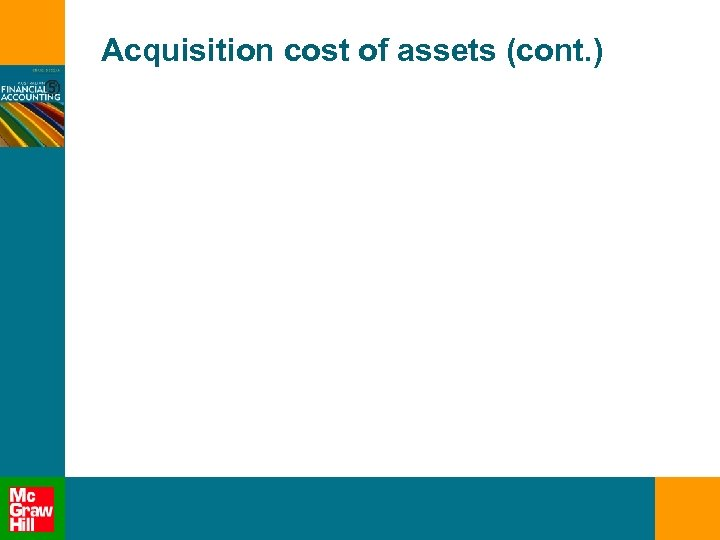Acquisition cost of assets (cont. )Acquisition cost of assets (cont. )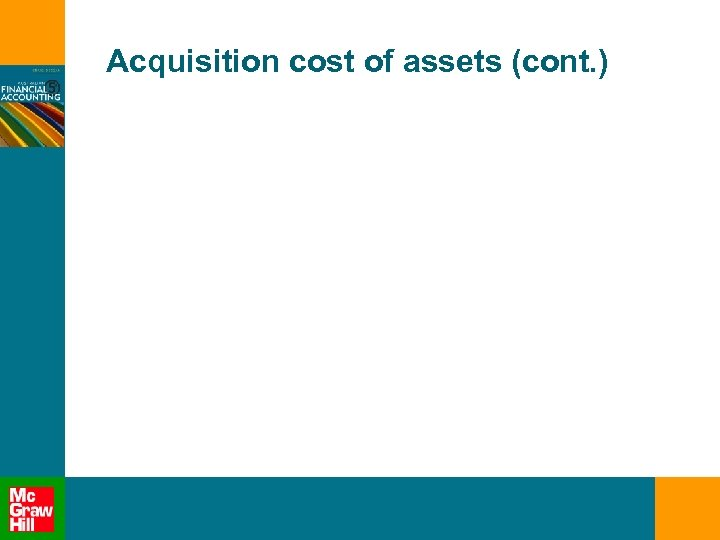Acquisition cost of assets (cont. )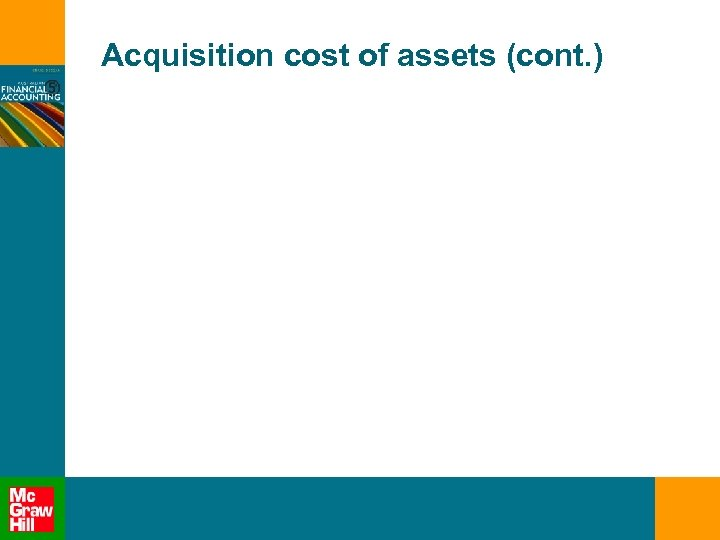Acquisition cost of assets (cont. )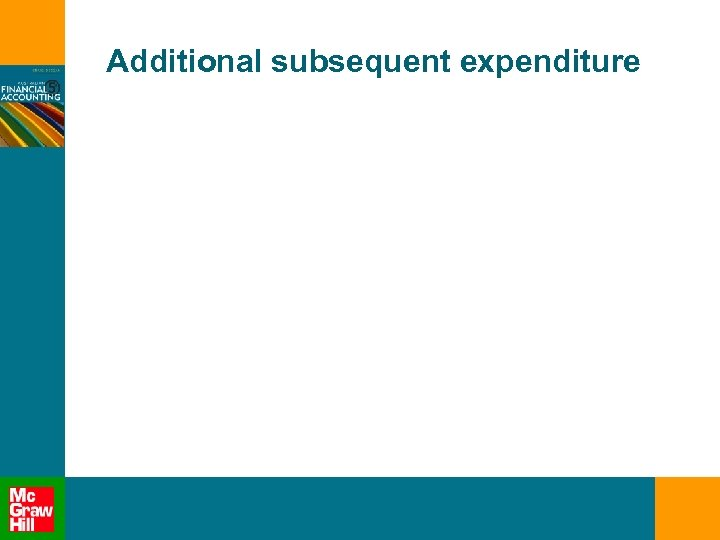Additional subsequent expenditure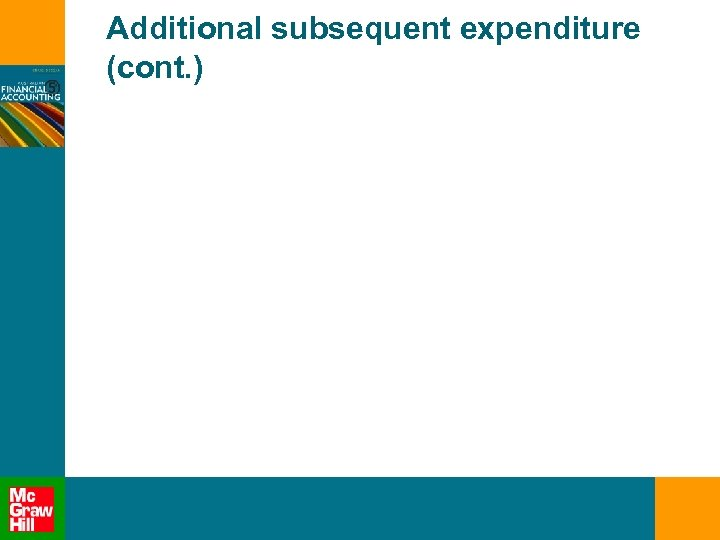Additional subsequent expenditure (cont. )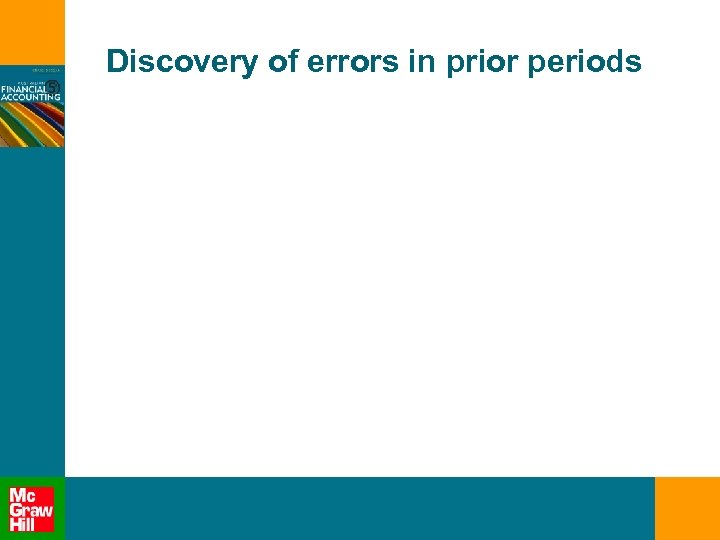Discovery of errors in prior periodsSummary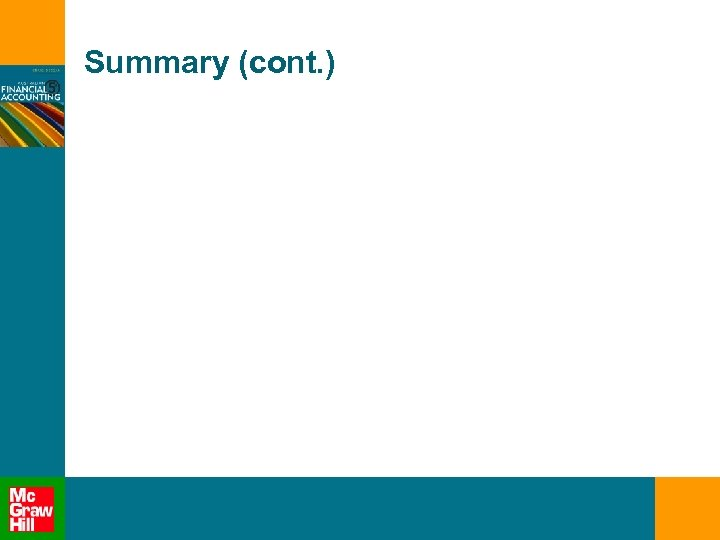Summary (cont. )